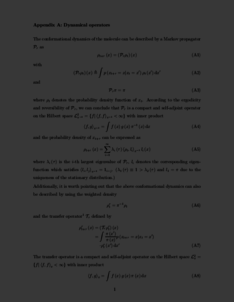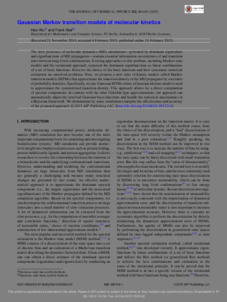Repository: Freie Universität Berlin, Math Department

# Gaussian Markov transition models of molecular kinetics

Wu, H. and Noé, F. (2015) Gaussian Markov transition models of molecular kinetics. J. Chem. Phys., 142 (8). 084104.Preview
PDF - Supplemental Material
172kBPreview

1MB

Official URL: http://dx.doi.org/10.1063/1.4913214

## Abstract

The slow processes of molecular dynamics (MD) simulations—governed by dominant eigenvalues and eigenfunctions of MD propagators—contain essential information on structures of and transition rates between long-lived conformations. Existing approaches to this problem, including Markov state models and the variational approach, represent the dominant eigenfunctions as linear combinations of a set of basis functions. However the choice of the basis functions and their systematic statistical estimation are unsolved problems. Here, we propose a new class of kinetic models called Markov transition models (MTMs) that approximate the transition density of the MD propagator by a mixture of probability densities. Specifically, we use Gaussian MTMs where a Gaussian mixture model is used to approximate the symmetrized transition density. This approach allows for a direct computation of spectral components. In contrast with the other Galerkin-type approximations, our approach can automatically adjust the involved Gaussian basis functions and handle the statistical uncertainties in a Bayesian framework. We demonstrate by some simulation examples the effectiveness and accuracy of the proposed approach.

Item Type: Article Physical SciencesMathematical and Computer Sciences Department of Mathematics and Computer Science > Institute of Mathematics > Comp. Molecular Biology 1503 BioComp Admin 29 Jan 2015 22:13 19 Jun 2017 13:23

Repository Staff Only: item control page